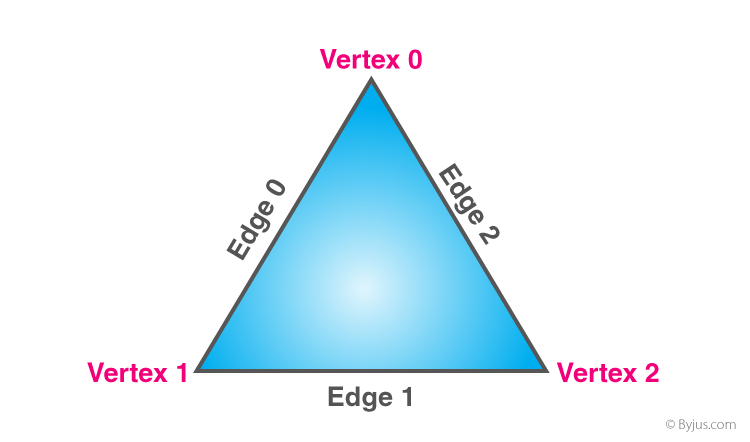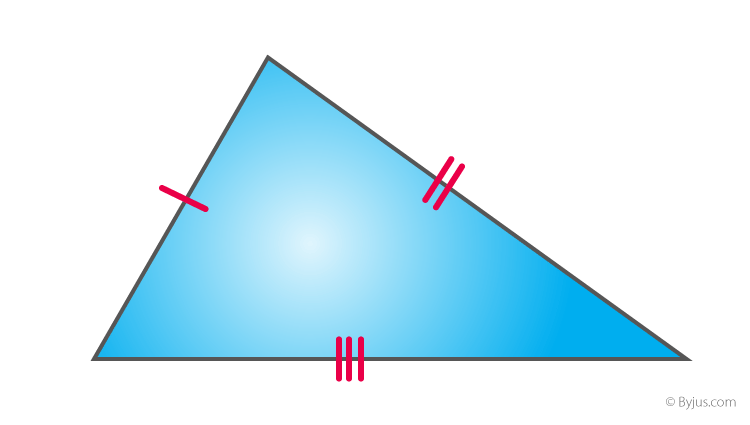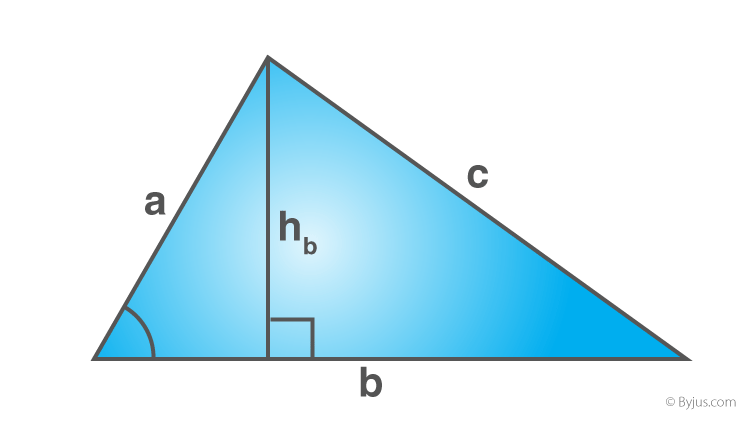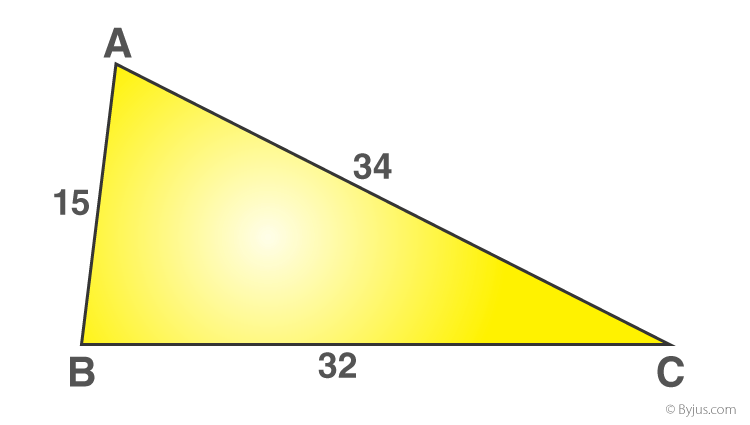# Scalene Triangle

Scalene Triangle is one of the types of triangles which is mentioned in geometry. We are going to discuss here its definition, formulas for perimeter and area and its properties. The triangles are defined based on its sides and angles. In geometry, a triangle is a closed two-dimensional plane figure with three sides and three angles and is shown as a three-sided polygon. It has three vertices and three edges.Based on the sides and the interior angles of a triangle, there are different types of triangle. According to the interior angles of the triangle, it can be classified into three types, namely:

• Acute Angle Triangle
• Right Angle Triangle
• Obtuse Angle Triangle

According to the sides of the triangle, the triangle can be classified into three types, namely;

• Scalene Triangle
• Isosceles Triangle
• Equilateral Triangle

## Scalene Triangle Definition

A scalene triangle is a triangle in which all the three sides are in different lengths, and all the three angles are of various measures. However, the sum of all the interior angles is always equal to 180 degrees.## Formulas

Let us learn the formulas to find the area and perimeter of a triangle which has unequal sides and angles, or we can say the scalene triangle.

### Area of a Scalene TriangleArea  = (1/2) x b x h square units

Where,

“b” refers to the base of the triangle

“h” refers to the height of the triangle

If the sides of the triangle are given, then apply the Heron’s formula.

Area of the triangle =  $A = \sqrt{s (s-a)(s-b)(s-c)}$ square units

Where, s is the semi perimeter of a triangle, which can be found using the formula

s = (a+b+c)/2

Here,

a, b, and c denotes the sides of the triangle

### Perimeter of a Scalene Triangle

The perimeter of a triangle is equal to the sum of the length of sides of a triangle and it is given as:

Perimeter  = a + b + c units

Example: Consider a given triangleTo find the perimeter for the given triangle, add the sides of a triangle

Therefore, perimeter = 15 + 34 + 32 = 81 cm

## Properties

Some of the important properties of the scalene triangle are as follows:

• It has no equal sides.
• It has no equal angles.
• It has no line of symmetry.
• It has no point symmetry.
• The angles inside this triangle can be an acute, obtuse or right angle.
• If all the angles of the triangle are less than 90 degrees(acute), then the centre of the circumscribing circle will lie inside a triangle.
• In a scalene obtuse triangle, the circumcenter will lie outside the triangle.

### Sample Problem

Question: Find the area of the scalene triangle ABC with the sides 8 cm, 6 cm and 4 cm

Solution:

Let a= 8 cm

b = 6 cm

c = 4 cm

If all the sides of a triangle are given, then use Heron’s formula.

Area of triangle =  $\sqrt{s (s-a)(s-b)(s-c)}$

Now, find the semiperimeter value

s = (a+b+c)/2

s = (8+6+4)/2

s = 18/2

s = 9

Now substitute the value of S in the area formula,

Area = $\sqrt{9(9-8)(9-6)(9-4)}$

$=\sqrt{9(1)(3)(5)}$

=√135

=11.6

Therefore, the area of the scalene triangle = 11.6 cm2

Download BYJU’S – The Learning App and learn all the Maths topics from personalised videos.

#### 1 Comment

1. DinahNkirote

Thanks for helping me to solve out my final exam will be great God bless you